# Fractal Indicator – Trading Strategies – 27 January 2022

Introduction to fractal indicator

The fractal indicator refers to a technical indicator that uses the fractal geometry found in the financial market. The Fractal Pattern Scanner is an advanced fractal indicator that offers the latest trading techniques after extensive research and development work on fractal geometry in the financial market. The most important feature of the fractal pattern scanner is the ability to measure tipping point probability as well as trend probability. Your ability to gauge the turning point probability and trend probability for each price movement can marginally enhance trading accuracy. Before we move on to the trading strategy with this fractal indicator, let’s cover in detail the turning point probability and trend probability.

Turning point probability and trend probability

In trading, tipping point probability can be viewed as the first attempt to determine price movement in a probabilistic manner. In science, it is the leading concept towards stochastic cycle modeling. Practically speaking, the method helps to determine the market timing of our trading by the quantitative method. In any cycle, when the body rises high enough, it must reach the top and must descend toward the bottom. Turning point probability applies the same principle to forex and stock market data. Hence, tipping point probability answers the question “How close is the price to the peak so that the price starts falling toward the bottom?” This is an important question to ask a trader because this expectation often helps us make money.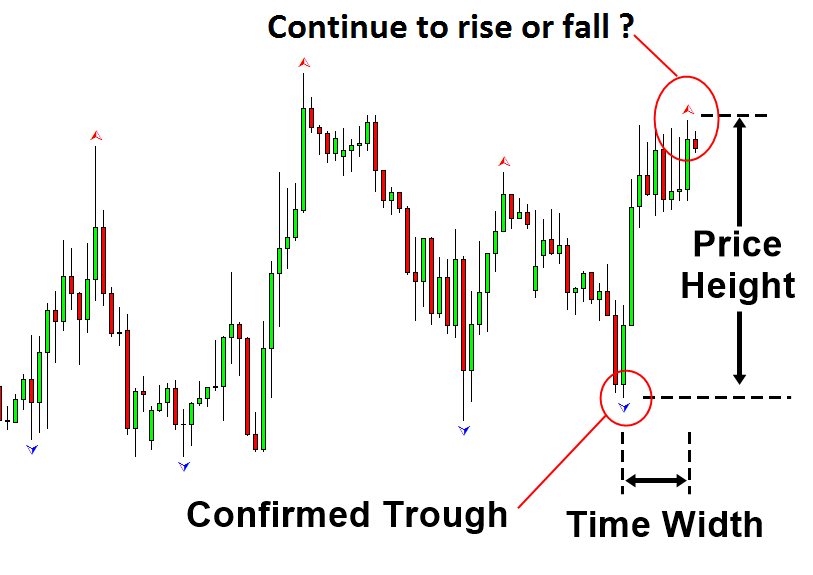Figure 4-16: Measurement of price height and time display in swing height

We cannot answer the question in an imperative sense. However, we can answer the question in a probabilistic sense. A higher turning point probability means that the price is close to the peak. So we have a great chance of seeing that price turn. For example, if the probability of the turning point is above 90%, then there is a high probability that the price will start declining near the current price.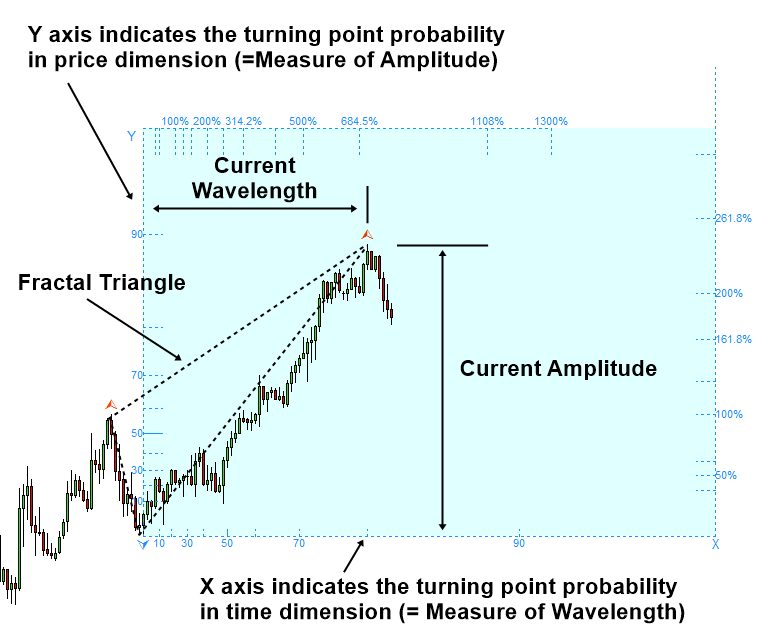Figure 4-17: Fibonacci probability graph measuring the probability of reaching a peak (www.algotrading-investment.com)

On the other hand, a lower probability of a tipping point means that the price is still far from the peak. Thus, there is a high chance that the price will continue to rise. For example, if the price is close to a turning point probability of 30%, then we can say that there is a high chance of the price going up. Of course, this is only true in a cyclical sense because the price will repeat endlessly up and down. However, this quantitative information is still useful for our trading. In fact, for convenience, one can use the turning point probability to get the trend probability. Simply put, you can subtract the turning point probability from 100% to get the trend probability.

Trend Probability = 100% – Turning Point Probability (i.e. only rule of thumb)

Hence, as a general rule, the 30% probability of the turning point is equal to the 70% (=100%-30%) probability of a trend where there is a high chance that the price will continue to rise in this case because it is far from the peak.Figure 4-18: Example of a 30% turning point probability (= 70% trend probability) for a peak

Support resistance with turning point probability and trend probability

The good thing is that tipping point probability goes well with most technical analysis. In the book “The Science of Support and Resistance, Fibonacci Analysis, Harmonic Pattern, Elliott Wave, and X3 Chart Pattern: In Forex Trading and the Stock Market,” we explain how to combine turning point probability with support and resistance. When you combine the turning point probability with support and resistance, the turning point probability helps you confirm the trend. For example, when the probability of a turning point is high at the support, you can expect the price to turn and rise at the support level.Figure 4-21: Turning point probably 82% at support on the H1 chart of the S&P 500

Similarly, when the probability of turning point is high at resistance, you can expect price to turn and fall at resistance. You can use more than 50% probability of turning point to confirm trading trend. However, usually, to predict the movement of the reversal, it is better to have a higher probability of the turning point. In this approach, the turning point probability complements the strength of support and resistance to predict the direction of the trade. This is a good trading strategy to avoid or reduce losing trades from false breakout and false reversal when using support and resistance. Similarly, in this book, we will show how to combine tipping point probability with supply and demand analysis to improve its performance. This will be a good guide to using probability for practical trading.Figure 4-22: The turning point is probably 55% at the resistance on the EURUSD H4 chart

Demand-to-supply analysis with tipping point probability and trend probability

The initial supply and demand zone refers to the area where the price has not been affected after determining the pattern for our trade. We can improve the accuracy of this initial supply and demand area by using tipping point probability. For example, when we trade with the demand zone, we can be sure that the probability of the turning point is high at the position of the buy price. A higher probability of a turning point indicates a higher chance of a price turning. In other words, this indicates the possibility of a price rally approaching the demand area soon. In the S&P 500 index example, the price at the first touch of the demand area was around a turning point probability of 70%. In addition to a higher time frame examination, the possibility of this turning point ensures that the price approaches the bottom before going up.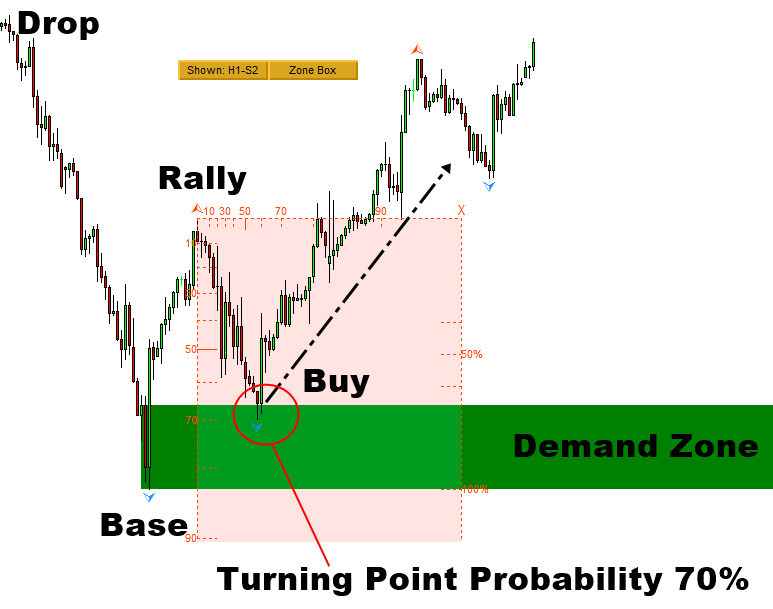Figure 8-1: S&P 500 H1 Time Frame

Let’s look at an example of a supply area trading. In the example of gold against the US dollar, the price at the first touch of the demand area was around the probability of a turning point of 50%. In practice, it is recommended to confirm the probability of a turning point of at least 50% before entering into buying or selling.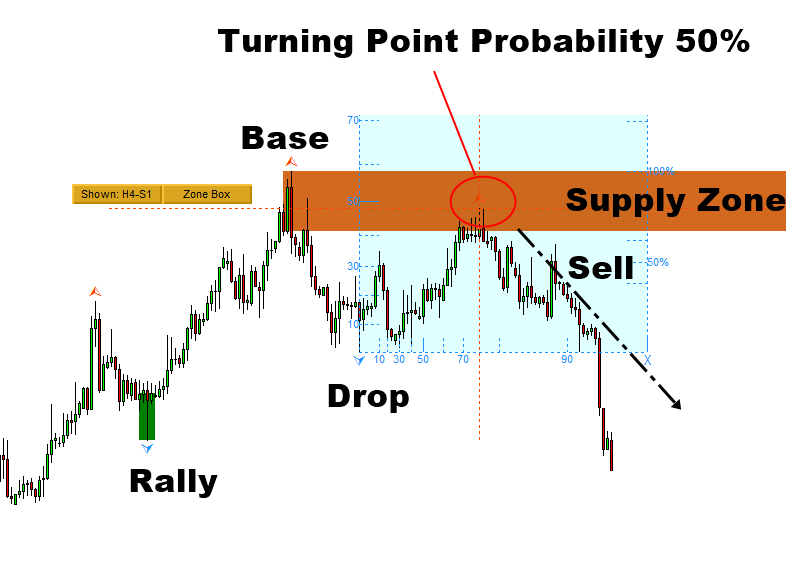Figure 8-2: Gold against the US dollar (= XAUUSD) H4 time frame (www.algotrading-investment.com)

We have provided some trading ideas using the Fractal Pattern Scanner with support and resistance analysis and demand/supply analysis. It was mainly for reverse trading examples. We chose these examples because support resistance and demand-supply analysis are easy to use and popular. This does not mean that the Fractal Pattern Scanner can only be used with these two technical analysis. In addition, this does not mean that the fractal pattern scanner can be used to detect a reversal trading opportunity alone. As long as you understand where to look for breakout trading and a reversal trading opportunity, you can combine the fractal pattern scanner with any other technical analysis for reversal and breakout trading. Below is a screenshot showing the basic information about the breakout and reversal trading opportunity. In particular, you can use any horizontal support and resistance with turning point probability and trend probability. With a little hands-on experience, you’ll soon learn how to control your success rate and do risk management with potentially tipping point.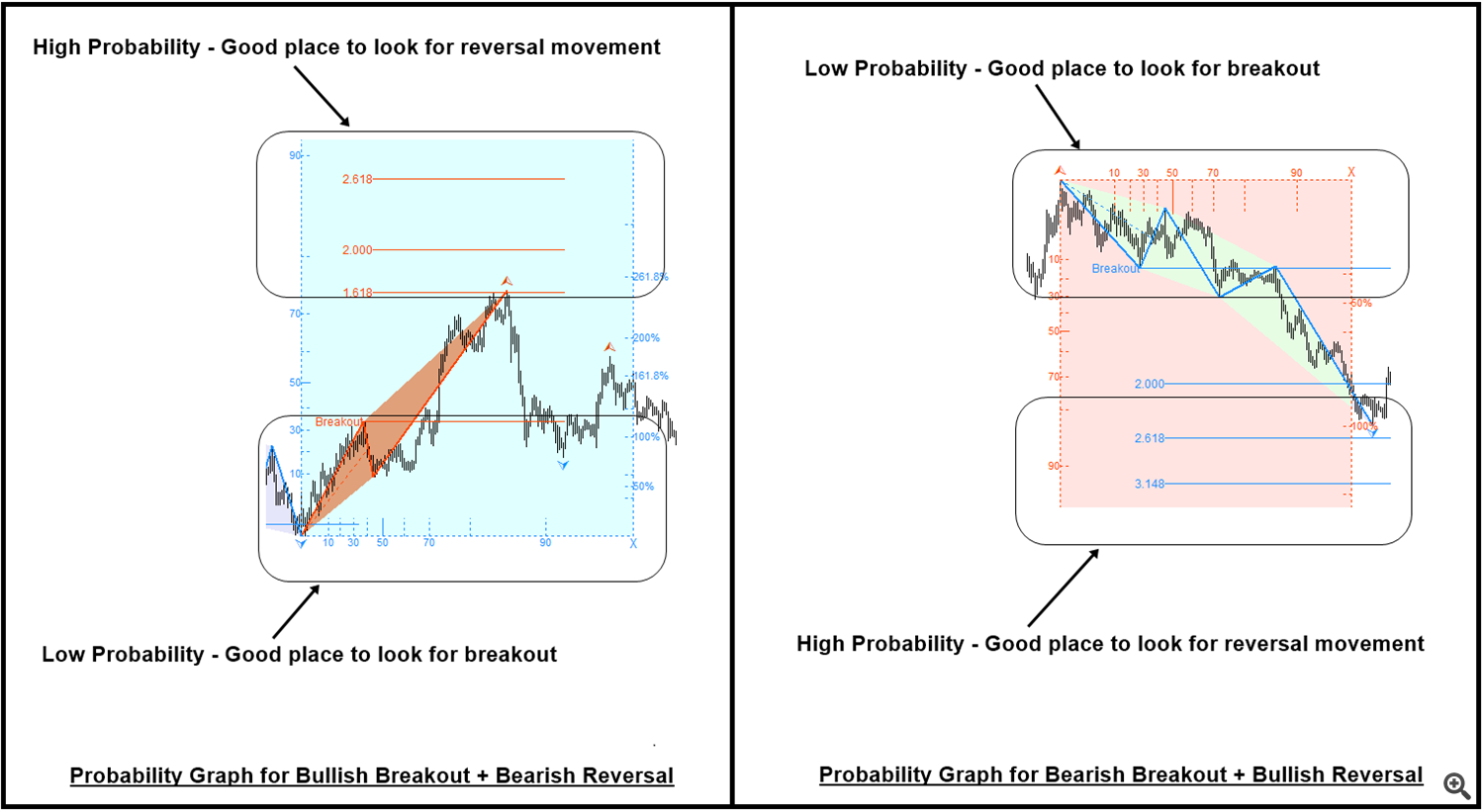You can find the fractal indicator for MetaTrader 4 from this link: https://www.mql5.com/en/market/product/49170

When using tipping point probability with a Harmonic Pattern or Elliott Wave Pattern, try to use the areas in the pattern completion interval to measure the relative strength of the breakout and reversal in the area.

You can find the fractal indicator for MetaTrader 5 from this link: https://www.mql5.com/en/market/product/49169

When using tipping point probability with a Harmonic Pattern or Elliott Wave Pattern, try to use the areas in the pattern completion interval to measure the relative strength of the breakout and reversal in the area.

Of course, the article has limited space to write while the book can provide more space and more organized ways to learn trading. Hence, we present the two books that provide you with the trading strategy in detail. If you want to become a successful trader, we recommend reading these two books.

Technical analysis in the forex and stock market

The science of support, resistance, Fibonacci analysis, harmonic pattern, Elliott wave and X3 chart pattern

If you would like to access free forex trading education, you can also visit our website here.

Summary of fractal indicator

Use the turning point probability and trend probability in this fractal indicator with any type of horizontal support and resistance to find a quick trade or a reversal trading opportunity.

1. Explanation of Breakout Pattern and Breakout Trading Signal: https://youtu.be/4XGuMIMaV6w

2. Turning support and resistance into a killer strategy using the Fractal Pattern Scanner:: https://youtu.be/UbORmOacKIQ

3. Trading with Fractal Wave and Stochastic Cycle with Fractal Pattern Scanner: https://youtu.be/_bstJrIevSQ

4. Gann Angles, Mother Wave Pattern, Fibonacci Extension, Andrew’s Fork and Probability Panels (Optional information only): https://youtu.be/QGHvKjECX1U

fractal style brochures

Understanding Gann Angles with Probability – A Whole New Technology

Combine Gann angles from multiple projection origins

Fractal Patterns Strategy Guides

Turning point, peak, trough, high swing, low swing, zigzag

Fractal wave, mother wave, child wave

Trading price pattern with potential turning point

Fibonacci probability chart

Mother and child wave with common probability

Anticipate volatility with tipping point probability

Trading support and resistance with potential turning point

Trading in a harmonic pattern with the potential for turning point

A falling wedge pattern and a rising wedge pattern with potential turning point

Gann angles with probability